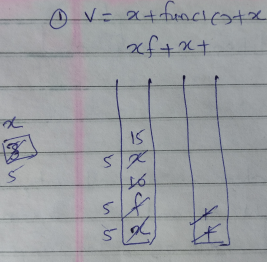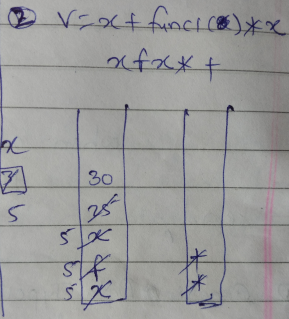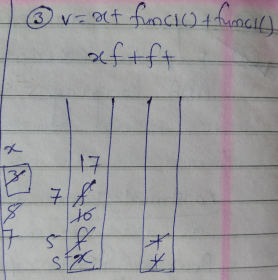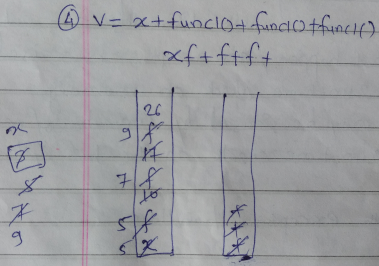## Saturday, 15 February 2020

### How C / C++ Compiler evaluates arithmetic expression / Side Effect of Global Variables in C / C++

In this post, we will see How C / C++ Compiler evaluates arithmetic expression / Side Effect of Global Variables in C / C++.

Check following program:

Program (sideeffect.cpp)
 #includeusing namespace std;int x=3,y=6;int func1(){x=x+2;return x;}int main(){int v;v=x+func1()+x;cout<<"v="<

In above example, many of us will have confusion over the output.
In this post, we will see how the arithmetic expression is evaluated with respect to this example.

Remember C / C++ compiler maintains two stacks: one for Operands and one for Operators. Expression is first converted to postfix expression.

1.In above example, xf+x+ is postfix expression where f stands for function func1().
Remember, whenever function is called, it get evaluated and it's return value is pushed to stack. While, for variables, current value is taken when operation is performed on it.

2.3.4.5.